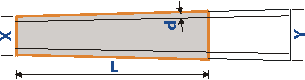Guitar building tips

# Calculating the fingerboard shape

The common way to determine the fretboard shape is to make a drawing on a large sheet of paper. Here is annother approach with the help of a calculator or a spreadsheet program.

Two measurements determine the width of the fretboard: The nut width X and Y the string spacing at the saddle (B) plus two times d ("d" is the distance from the outer strings to the fingerboard edge which should be equal along the entire fretboard length).You can calculate the width of the fretboard at any length (L):

```
L ( Y - X )
WIDTH = X +  -----------
S

d ... distance of outer strings
to fingerboard edge
X ... nut width (A + 2d)
Y ... bridge stringspread (B) + 2d
L ... distance from nut
S ... scale length
```

With the formula shown above you can for instance calculate the width at the 23rd fret (the end of the fretboard on a 22-frets guitar) which gives you all the necessary data for drawing the fingerboard outline:

For instance:

Width at nut (X) = 42mm (1-5/8")

Distance nut to 23rd fret (L) = 462.14mm (18.195")

d = 3.5mm (1/8")

String spread at the bridge (B) = 54mm (2-1/8")

Y = B + 2d

Width at 23rd fret = 55.97mm (2.203")

There's a fretboard shape calculator available